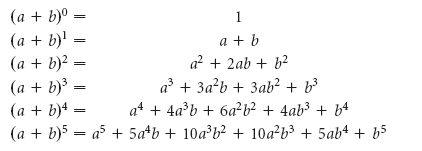Request a Tool

Pascal Triangle Binomial Expansion Calculator

Pascal Triangle Binomial Expansion Calculator is use to calculate pascal triangle from input number of rows.

Output

Pascal triangle binomial expansion
0

Formula• (a+b)^0 = for row 1
• (a+b)^1 = for row 2
• (a+b)^2 = for row 3
• (a+b)^3 = for row 4
• (a+b)^4 = for row 5
• (a+b)^5 = for row 6

Defination / Uses

The coefficients for an expanded binomial of the form (a + b)n, where n is the triangle's row, can be found using Pascal's Triangle.

Welcome to our Pascal's triangle calculator, where you will discover both how to use and why you should use it in the first place. Don't worry, unlike a typical triangle, this concept doesn't necessitate any area formulas or unit computations. So, what exactly is Pascal's triangle? Well, it's a fun method to view binomial expansion and calculate the number of combinations. But, before we go into the details of Pascal's triangle patterns, let's go through the fundamentals. Pascal's triangle is an equilateral triangle-shaped table of numbers in which the k-th number in the n-th row indicates how many permutations of k elements there are from a set of n elements.